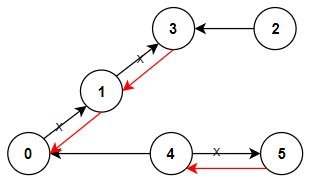2020-05-31

## 1466. Reorder Routes to Make All Paths Lead to the City Zero

### Question:

There are `n` cities numbered from `0` to `n-1` and `n-1` roads such that there is only one way to travel between two different cities (this network form a tree). Last year, The ministry of transport decided to orient the roads in one direction because they are too narrow.

Roads are represented by `connections` where `connections[i] = [a, b]` represents a road from city `a` to `b`.

This year, there will be a big event in the capital (city 0), and many people want to travel to this city.

Your task consists of reorienting some roads such that each city can visit the city 0. Return the minimum number of edges changed.

It’s guaranteed that each city can reach the city 0 after reorder.

#### Example 1:``````Input: n = 6, connections = [[0,1],[1,3],[2,3],[4,0],[4,5]]
Output: 3
Explanation: Change the direction of edges show in red such that each node can reach the node 0 (capital).
``````

#### Example 2:``````Input: n = 5, connections = [[1,0],[1,2],[3,2],[3,4]]
Output: 2
Explanation: Change the direction of edges show in red such that each node can reach the node 0 (capital).
``````

#### Example 3:

``````Input: n = 3, connections = [[1,0],[2,0]]
Output: 0
``````

Constraints:

• `2 <= n <= 5 * 10^4`
• `connections.length == n-1`
• `connections[i].length == 2`
• `0 <= connections[i], connections[i] <= n-1`
• `connections[i] != connections[i]`

### Solution:

Using BFS to traverse the tree and check if the path is an out path.

``````class Solution {
public int minReorder(int n, int[][] connections) {
HashMap<Integer, Set<Integer>> outmap = new HashMap<>();
HashMap<Integer, Set<Integer>> map = new HashMap<>();
Queue<Integer> que = new LinkedList<>();
boolean[] visited = new boolean[n];
int counter = 0;

for (int[] i : connections) {
int from = i;
int to = i;
map.computeIfAbsent(from, k -> new HashSet<Integer>());
map.computeIfAbsent(to, k -> new HashSet<Integer>());
outmap.computeIfAbsent(from, k -> new HashSet<Integer>());

}

visited = true;

while(!que.isEmpty()) {
int curr = que.poll();
for (int next : map.getOrDefault(curr, new HashSet<>())) {
if (visited[next] == true) continue;
visited[next] = true;
if (outmap.get(curr) != null && outmap.get(curr).contains(next)) {
counter++;
}